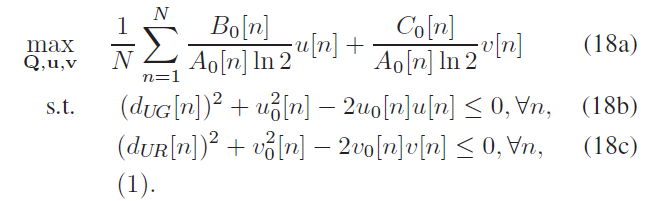# How to do a quadratic form to a log-convex scalar value?

The main aim here is to optimize the trajectory of UAV for 3-time slots. Since I know the initial position of UAV at the beginning, I manually brought the distances (U, V) at the first time slot, but I want to optimize the (U, V) to bring me the maximum OBJ. while having quadratic constraints. the problem is the error that appears
incorrect log-convex - log-convex, Is there any way to have a quadratic function consisting of log-convex value?
I tried to use a quad_form and Sum_Square functions, but an error appears saying that I need an affine expression.

Here is my code for clarification:
cvx_begin
variable Qx
variable Qy
OBJ=0;
U=sqrt((UAV_horizontal(1,1)-User_Place(1,1))^2+(UAV_horizontal(2,1)-User_Place(2,1))^2+(80)^2);
V=sqrt((UAV_horizontal(1,1))^2+(UAV_horizontal(2,1))^2+(80-40)^2);
OBJ=OBJ+((Bo/(Ao*0.693)U)+(Co/(Ao0.693))V);
Qx_new=UAV_horizontal(1,1);
Qy_new=UAV_horizontal(2,1);
for i=2:3
Qx_old=Qx_new;
Qy_old=Qy_new;
OBJ=((Bo/(Ao
0.693))norm([80 power(2,norm([Qx Qy-70]))])+(Co/(Ao0.693))*norm([40 power(2,norm([Qx Qy]))]));
Qx_new=Qx;
Qy_new=Qy;
U=norm([80 power(2,norm([Qx_new Qy_new-70]))]);
V=norm([40 power(2,norm([Qx_new Qy_new]))])

subject to
square(U)+square(Uo)-(2UoU)<=0;
square(V)+square(Vo)-(2VoV)<=0;
power (2,norm([Qx_new-Qx_old Qy_new-Qy_old]))<=25;

end
maximize(sum(OBJ(:)));
cvx end

There is no `log` found in your code and I don’t know what you meaned. Please show the mathematical function you want to express and prove its convexity. As for your code, the OBJ should be defined as `expression OBJ` before it is used; The `maximize(sum(OBJ(:)));` might be better placed before `subject to`.

Here is the full code including Ao, Bo, Co. clc
clear all
Ao=1;
Bo=-1;
Co=-10;
Uo=76.8115
Vo=40;
UAV_horizontal=zeros(2,740);
UAV_horizontal(:,1)=[-500;20];
%Placement of Ground User
User_Place=[0,70].’;

%RIS Values
RIS_Place=[0,0].’;

cvx_begin
variable Qx
variable Qy
OBJ=0;
U=sqrt((UAV_horizontal(1,1)-User_Place(1,1))^2+(UAV_horizontal(2,1)-User_Place(2,1))^2+(80)^2);
V=sqrt((UAV_horizontal(1,1))^2+(UAV_horizontal(2,1))^2+(80-40)^2);
OBJ=OBJ+((Bo/(Ao0.693)U)+(Co/(Ao0.693))V);
Qx_new=UAV_horizontal(1,1);
Qy_new=UAV_horizontal(2,1);
for i=2:3
Qx_old=Qx_new;
Qy_old=Qy_new;
OBJ=((Bo/(Ao
0.693))norm([80 power(2,norm([Qx Qy-70]))])+(Co/(Ao0.693))norm([40 power(2,norm([Qx Qy]))]));
Qx_new=Qx
Sq_Qx_new=norm(Qx)
Qy_new=Qy;
U=norm([80 power(2,norm([Qx_new Qy_new-70]))]);
V=norm([40 power(2,norm([Qx_new Qy_new]))])
maximize(sum(OBJ(:)));
subject to
%sum_square(U-Uo)<=0;
square(U)+square(Uo)-(2
Uo
U)<=0;
square(V)+square(Vo)-(2VoV)<=0;
power (2,norm([Qx_new-Qx_old Qy_new-Qy_old]))<=25;

end
cvx_end
and also here is the mathematical problem that I try to optimizereplacing every Dug [n] with U,
and every Dur[n] with V.

I searched the code to see how the model contains a log function, but I can’t find any. The thing is when I run the code, he considers that this model will be solved by a successive approximation method

Your code is not reproducible. Besides, please use a Markdown format to write it. and Show all the output(the error message,etc.), please.